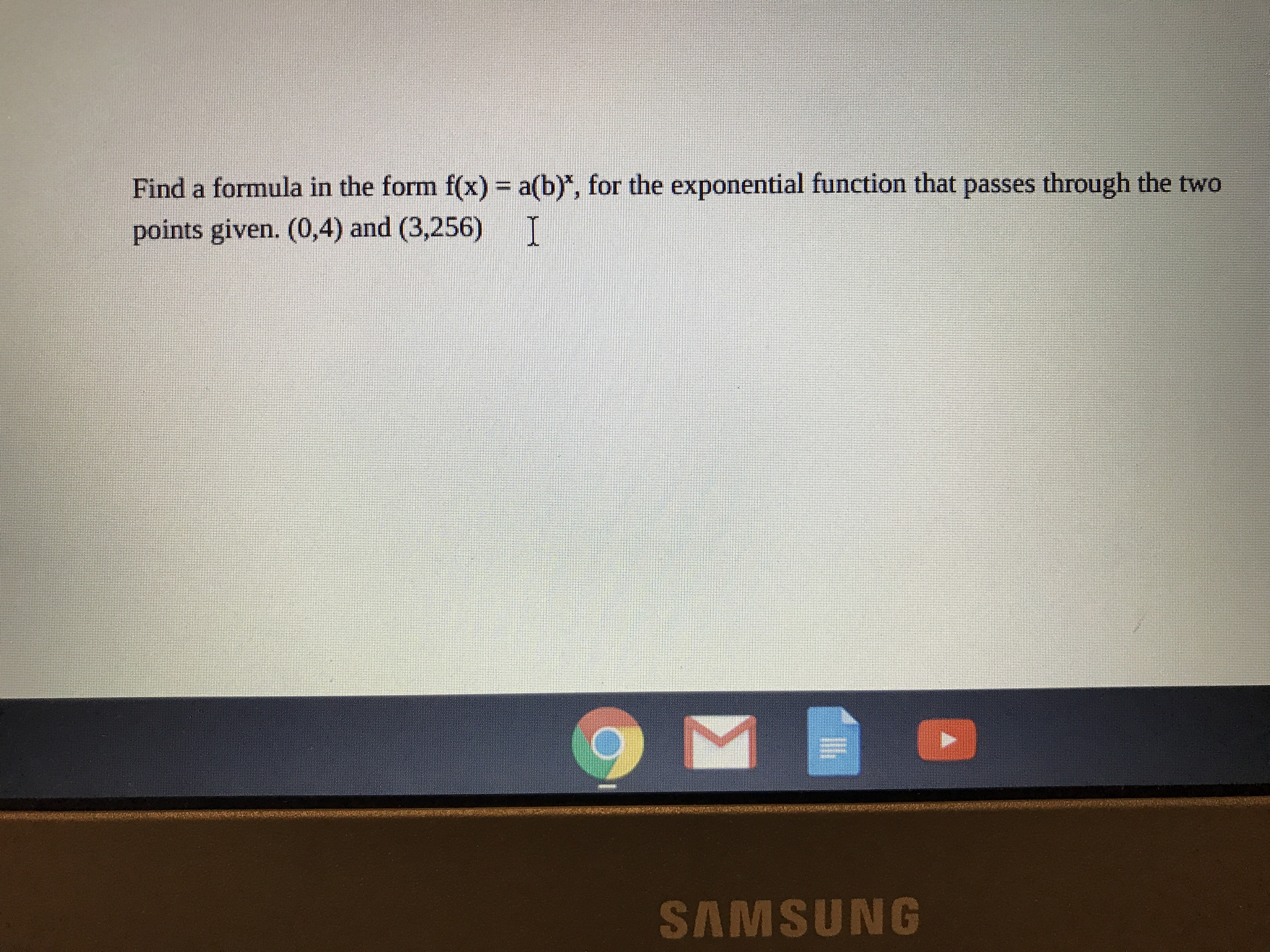# Find a formula in the form f(x) = a(b)", for the exponential function that passes through the two points given. (0,4) and (3,256) I SAMSUNG

Questionhelp_outlineImage TranscriptioncloseFind a formula in the form f(x) = a(b)", for the exponential function that passes through the two points given. (0,4) and (3,256) I SAMSUNG fullscreen

### Want to see this answer and more?

Experts are waiting 24/7 to provide step-by-step solutions in as fast as 30 minutes!*

*Response times vary by subject and question complexity. Median response time is 34 minutes and may be longer for new subjects.
Tagged in
MathCalculus

### Other